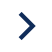GlossaryGlossaryReturn On Investment (ROI)
Return On Investment (ROI)
Return on investment (ROI) is a metric to measure the profitability of an investment by dividing the net profit by the original cost of the investment, expressed as a percentage.

## What is ROI?

ROI stands for "Return on Investment," and it is a financial measure that calculates the profitability of an investment. The higher the ROI, the more profitable the investment. ROI is a helpful tool for evaluating the efficiency of an investment and can be used to compare the profitability of different investments. It is important to note that ROI does not take into account the length of time that an investment is held and is best used to compare investments that are held for similar periods of time.

## How do you calculate ROI?

ROI can be calculated by dividing the net profit of the investment by the original cost of the investment. Below is the formula for calculating ROI.

ROI = (Net Profit of Investment / Original Cost) x 100

To calculate ROI, you first need to determine the net profit of the investment. The net profit of investment can be determined by subtracting the original cost of the investment from the total revenue generated by the investment. Once you have the net profit, you can divide that number by the original cost of the investment and then multiply the result by 100 to express it as a percentage.

For example, if you invested \$100 in a promotion and it generated a profit of \$130, the ROI would be calculated as follows: ROI = (\$130-\$100 / \$100) x 100 = 30%

### How do you calculate the ROI of an app?

To calculate the net profit of the app, you will need to consider the total revenue generated by the app and subtract any operating expenses, such as the cost of development, marketing, and hosting. The original cost of the app would be the total amount of money invested in its development and launch.

It's important to note that calculating the ROI of an app can be more complex than calculating the ROI of some other types of investments because several variables can affect an app's profitability. For example, the revenue generated by an app may depend on a number of factors, such as the number of downloads, the price of the app, and the effectiveness of in-app purchases or advertising. Additionally, the operating expenses of an app could vary over time, so it may be necessary to recalculate the ROI on a regular basis to get an accurate picture of the app's profitability.

It can also be helpful to consider the lifetime value (LTV) of an app's users, which is the total value that a user is expected to generate throughout their relationship with the app. Calculating the LTV can help you understand an app's long-term profitability and make more informed decisions about how to allocate resources to maximize ROI.

## Why is ROI important to marketers?

ROI is an important metric to marketers because it allows them to understand the profitability of their marketing campaigns and compare the relative efficiency of different marketing strategies. By calculating the ROI of a marketing campaign, a marketer can determine how much revenue was generated in relation to the amount of money spent. With this information, marketers can make informed decisions about where to allocate their resources and identify marketing strategies that are likely to be more or less successful.

For example, if a campaign has a high ROI, it generates a lot of revenue relative to the amount of money spent on it. Therefore, a high ROI might indicate that the campaign is effective and is a good use of the marketing budget. On the other hand, if a marketing campaign has a low ROI, it may be an indication that the campaign is not generating much revenue relative to the amount of money being spent.

Subscribe to the newsletter for marketing trends, insights, and strategies.
Get a mail whenever a new article is uploaded.